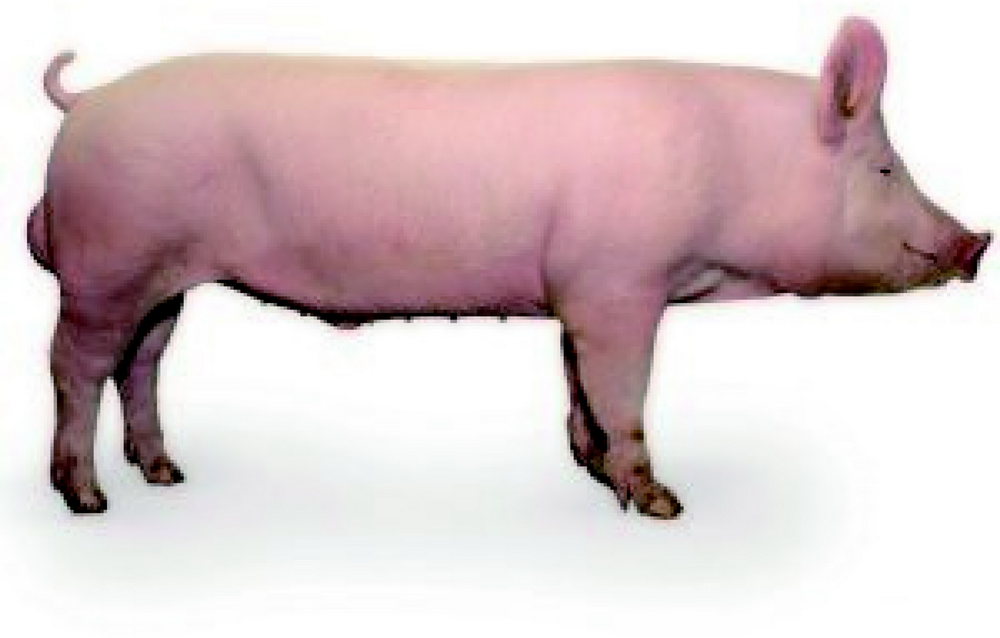# AI 安全之对抗样本入门 (37)：打造对抗样本工具箱 3.6&3.7(使用预训练模型)

• ResNet50
• VGG16
• InceptionV3

from IPython.display import Image,displaypath = "../picture/pig.jpg"display(Image(filename=path))
1. 使用 Keras 进行图片分类

Keras 的应用模块 Application 提供了带有预训练权重的 Keras 模型，这些模型可以用来进行预测、特征提取。模型的预训练权重将下载到~/.keras/models/ 并在载入模型时自动载入。from keras.applications.resnet50 import ResNet50from keras.preprocessing import imagefrom keras.applications.resnet50 import preprocess_input, decode_predictionsimport numpy as npmodel = ResNet50(weights='imagenet')img_path = pathimg = image.load_img(img_path, target_size=(224, 224))x = image.img_to_array(img)x = np.expand_dims(x, axis=0)x = preprocess_input(x)

preds = model.predict(x)print('Predicted:', decode_predictions(preds, top=3))Predicted:[('n02395406', 'hog', 0.98398596),('n02396427', 'wild_boar', 0.0074134255),('n03935335', 'piggy_bank', 0.006954492)]
1. 使用 PyTorch 进行图片分类

PyTorch 通过 torchvision 库封装了对预训练模型的下载和使用，这些模型可以用来进行预测、特征提取。模型的预训练权重将下载到~/.torch/models/ 并在载入模型时自动载入。

import osimport numpy as npimport torchimport torch.nnimport torchvision.models as modelsfrom torch.autograd import Variableimport torch.cudaimport torchvision.transforms as transformsfrom PIL import Image#手工调用 eval 方法进入预测模式resnet50=models.resnet50(pretrained=True).eval()img=Image.open(path)img=img.resize((224,224))img = np.array(img).copy().astype(np.float32)

mean = [0.485, 0.456, 0.406]std = [0.229, 0.224, 0.225]img /= 255.0img = (img - mean) / stdimg = img.transpose(2, 0, 1)img=np.expand_dims(img, axis=0)img = Variable(torch.from_numpy(img).float())

label=np.argmax(resnet50(img).data.cpu().numpy())print("label={}".format(label))label=341

https://s3.amazonaws.com/deep-learning-models/image-models/imagenet_class_index.json

print('Predicted:', decode_predictions(resnet50(img).data.cpu().numpy(),top=3))Predicted:[('n02395406', 'hog', 15.661886),('n02396427', 'wild_boar', 10.353137),('n03935335', 'piggy_bank', 10.098382)]
1. 使用 MXNet 进行图片分类

MXNet 通过 gluon 库封装了对预训练模型的下载和使用，这些模型可以用来进行预测、特征提取。模型的预训练权重将下载到~/.mxnet/models/ 并在载入模型时自动载入，更多模型可以参考以下链接。

http://mxnet.incubator.apache.org/versions/master/api/python/gluon/model_zoo.html

from mxnet import gluonimport mxnet as mxfrom mxnet.gluon import nnfrom mxnet import ndarray as ndfrom mxnet import autogradimport numpy as npresnet=mx.gluon.model_zoo.vision.resnet50_v2(pretrained=True)img=Image.open(path)img=img.resize((224,224))img = np.array(img).copy().astype(np.float32)

MXNet 对图像的预处理与 Pytorch 一样需要手工进行。

mean = [0.485, 0.456, 0.406]std = [0.229, 0.224, 0.225]img /= 255.0img = (img - mean) / stdimg = img.transpose(2, 0, 1)

img=np.expand_dims(img, axis=0)array = mx.nd.array(img)outputs=resnet(array).asnumpy()label = np.argmax(outputs)print(label)
1. 使用 TensorFlow 进行图片分类

TensorFlow 的模型多以 pb 文件形式保存。以 Inception 为例，模型文件为 pb 格式，其中的 classify_image_graph_def.pb 文件就是训练好的 Inception 模型，imagenet_synset_to_human_label_map.txt 是类别文件。

wgethttp://download.tensorflow.org/models/image/imagenet/inception-2015-12-05.tgztar -zxvf inception-2015-12-05.tgzx classify_image_graph_def.pbx cropped_panda.jpgx imagenet_2012_challenge_label_map_proto.pbtxtx imagenet_synset_to_human_label_map.txtx LICENSE

path = "../picture/pig.jpg"image_data = tf.gfile.FastGFile(path, "rb").read()

session=tf.Session()def create_graph(dirname):    with tf.gfile.FastGFile(dirname, 'rb') as f:        graph_def = session.graph_def        graph_def.ParseFromString(f.read())        _ = tf.import_graph_def(graph_def, name='')create_graph("models/classify_image_graph_def.pb")session.run(tf.global_variables_initializer())

logits=session.graph.get_tensor_by_name('softmax/logits:0')x = session.graph.get_tensor_by_name('DecodeJpeg/contents:0')predictions=session.run(logits,feed_dict={x:image_data})predictions = np.squeeze(predictions)top_k = predictions.argsort()[-3:][::-1]for node_id in top_k:      human_string = node_lookup.id_to_string(node_id)      score = predictions[node_id]      print('%s (score = %.5f)' % (human_string, score))

tensorlist=[n.name for n in session.graph_def.node]print(tensorlist)

TensorFlow 还提供了大量 ckpt 格式的预训练模型，也需要手工下载后加载使用。ckpt 文件相当于只保存了网络的参数，如果要完整使用，需要自行定义网络的结构。

wget http://download.tensorflow.org/models/resnet_v2_50_2017_04_14.tar.gztar -zxvf resnet_v2_50_2017_04_14.tar.gz

TensorFlow 提供了大量工具函数便于使用预训练模型，需要单独安装并设置到系统路径。

git clone https://github.com/tensorflow/models/

sys.path.append("models/models/research/slim/")

TensorFlow 通过 slim 定义了大量常见的网络结构，以 resnet 为例，就在 slim/nets/resnet_v2 完成了其网络定义。

from nets import resnet_v2

slim 对数据预处理以及归一化做了封装，可以直接调用相应的 API 完成。TensorFlow 的预训练模型在预测时需要显式指定 is_training=False 来关闭反向传递。

path = "../picture/pig.jpg"image = tf.image.decode_jpeg(tf.gfile.FastGFile(path,'rb').read(), channels=3)image_size = resnet_v2_50.default_image_sizeprocessed_image = inception_preprocessing.preprocess_image(image, image_size,image_size, is_training=False)processed_images  = tf.expand_dims(processed_image, 0)

with slim.arg_scope(resnet_v2.resnet_arg_scope()):    logits, _ = resnet_v2.resnet_v2_50(processed_images, num_classes=1001,is_training=False)probabilities = tf.nn.softmax(logits)saver=tf.train.Saver()with tf.Session() as sess:     saver.restore(sess,'models/resnet_v2_50/resnet_v2_50.ckpt')

np_image, probabilities = sess.run([image, probabilities])probabilities = probabilities[0, 0:]sorted_inds = [i for i in sorted(enumerate(-probabilities), key=lambda x:x)]

Probability 99.08% => [hog, pig, grunter, squealer, Sus scrofa]Probability 0.84% => [piggy bank, penny bank]Probability 0.03% => [wild boar, boar, Sus scrofa]Probability 0.01% => [French bulldog]Probability 0.01% => [hippopotamus, hippo, river horse, Hippopotamus amphibius]

names = imagenet.create_readable_names_for_imagenet_labels()for i in range(5):    index = sorted_inds[i]print('Probability %0.2f%% => [%s]'                  % (probabilities[index] * 100,  names[index]))

(本章小结)